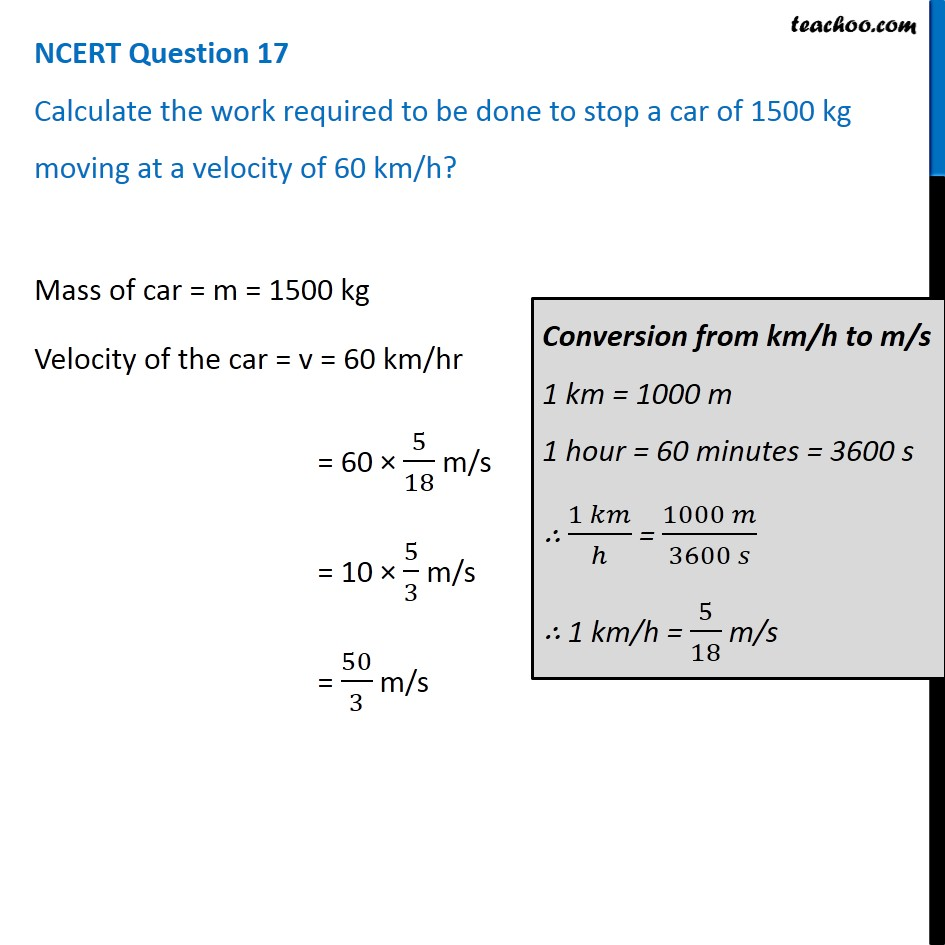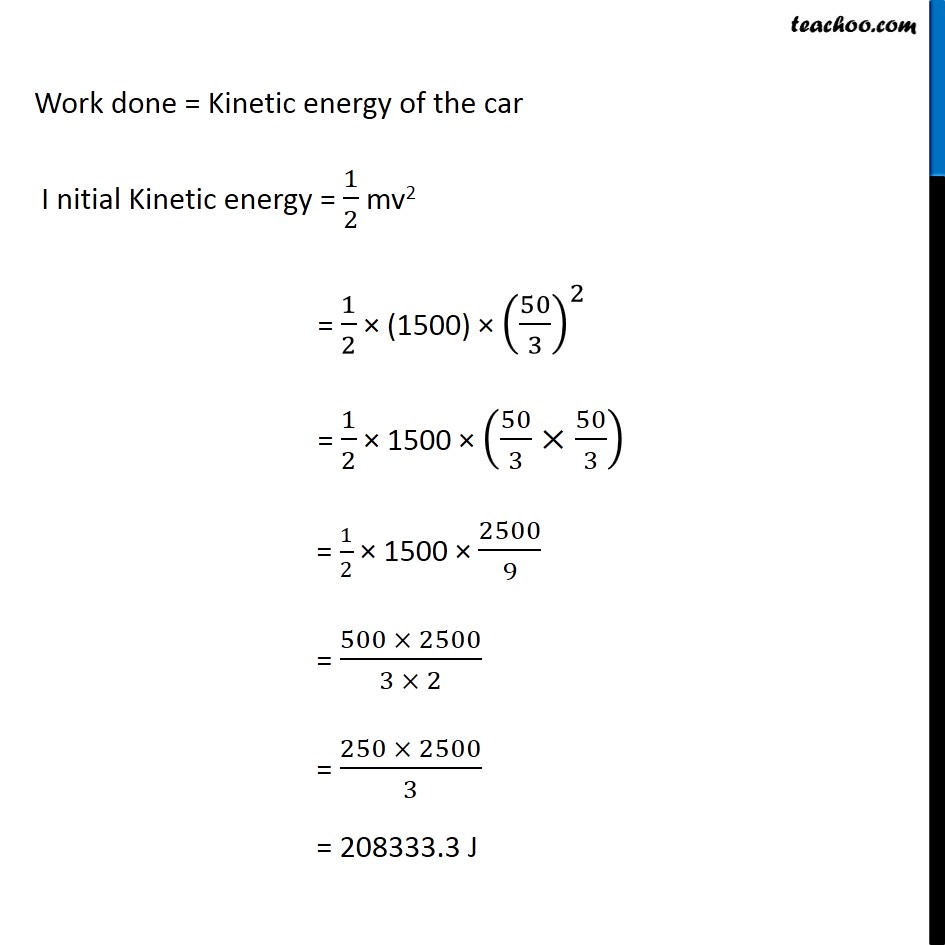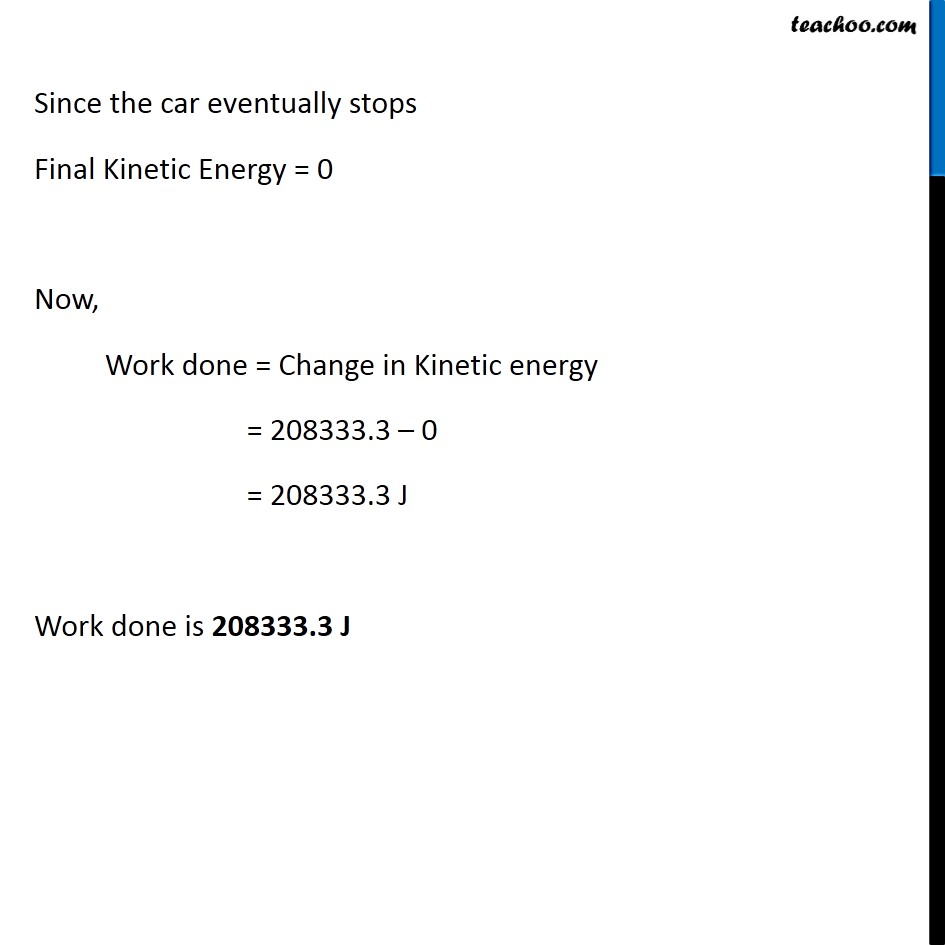NCERT Questions

Class 9
Chapter 11 Class 9 - Work and EnergyLearn in your speed, with individual attention - Teachoo Maths 1-on-1 Class

### Transcript

NCERT Question 17 Calculate the work required to be done to stop a car of 1500 kg moving at a velocity of 60 km/h? Mass of car = m = 1500 kg Velocity of the car = v = 60 km/hr = 60 × 5/18 m/s = 10 × 5/3 m/s = 50/3 m/s Conversion from km/h to m/s 1 km = 1000 m 1 hour = 60 minutes = 3600 s ∴ (1 𝑘𝑚)/ℎ = (1000 𝑚)/(3600 𝑠) ∴ 1 km/h = 5/18 m/s Work done = Kinetic energy of the car I nitial Kinetic energy = 1/2 mv2 = 1/2 × (1500) × (50/3)^2 = 1/2 × 1500 × (50/3×50/3) = 1/2 × 1500 × 2500/9 = (500 × 2500)/(3 × 2) = (250 × 2500)/3 = 208333.3 J Since the car eventually stops Final Kinetic Energy = 0 Now, Work done = Change in Kinetic energy = 208333.3 – 0 = 208333.3 J Work done is 208333.3 J